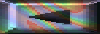BEM simulation of ellipsoid HRTFs
 ....some important details......

By Yuvi Kahana – May 99

 Front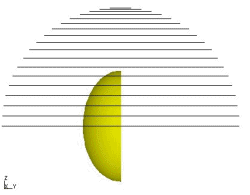Top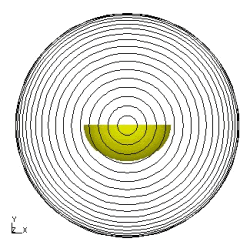Side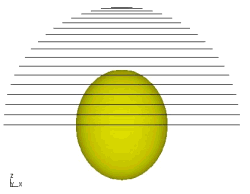Isometric view of field points  (72 in each circle)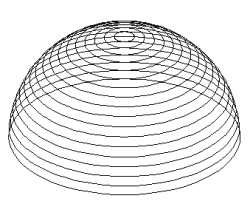Why?

• Better geometry match to the human head than a sphere
• No analytical solution exists (yet). If you have an access to such a solution  I'll be happy to know about it !
• Will be used for research on the superposition of various components for HRTFs such as the pinna (simulated on a baffle), torso.
• Will be used for psychoacoustic investigation of elevation ques.
• I'll be happy to hear about more/different reasons why I did this work....

Dimensions

• Approximation to an ellipsoid - rx=9.6, ry=7.9, rz=11.6 [cm].  This was found by stretching the axes of a sphere to match the dimensions of the CORTEX dummy-head.
• The ears were positioned as close as possible to the original positions of the ears of the CORTEX dummy-head. The positions that were found : 100.220  and 259.780 for the right and left ear, respectively (00 is in the front). These were positioned 0.86 [cm] below (in the z-axis) the origin of the ellipsoid.
Convention of angles
• Elevation - 00  at the level of the origin of the ellipsoid ('eye' level), 900 above the head
• Azimuth - 00 exactly in the front, 900 to the right, with respect to the origin of the ellipsoid, 1800 - behind, 2700 to the left.

So what have we got here?

• The frequency response ('HRTFs') at the positions of the 'ears' of an ellipsoid from DC up to 5kHz in steps of 100 Hz for three distances: 25 cm from the centre of the ellipsoid, 1m, and 10 m. The volume velocity of the monopole was constant and produced 1 Pa at 1m (power of 1.50857e-02 W). The data is in the form of complex numbers.
• The frequency response of a perfect monopole where the 'microphone' was positioned at the centre of the ellipsoid.
• All the calculation were carried out in steps of 5 deg. NO INTERPOLATION WERE MADE IN ANY STAGE.
• In total there are
• 19 elevation angles (00 to 900 )
• 72 azimuth angles (00 to 3550 )
• 51 frequencies (1 [Hz], 100 [Hz], 200 [Hz],...5000 [Hz])
• 3 distances (25 [cm], 1 [m], and 10 [m] away from the centre of the ellipsoid)
• Total:
• 4104 HRTFs
• 209,304 accurate simulation at single frequencies !
 What is inside this Matlab workspace? ellips_hrtf.mat includes the following matrices  source_10m   51x3  double array (freq,real,imag)  source_1m     51x3  double array (freq,real,imag)  source_25cm 51x3  double array (freq,real,imag)  tot_fin_ellips_10m 19x72x51  double array (complex) (elevation,azimuth,response)  tot_fin_ellips_1m 19x72x51   double array (complex) (elevation,azimuth,response)  tot_fin_ellips_25cm 19x72x51 double array (complex) (elevation,azimuth,response)  other m, html and gif filesThe method

• The direct method of BEM was used using the principle of reciprocity. This produced negligible errors. 15 over-determination points were used to remove irregular frequencies. Due to symmetries a 'rule of thumb' with 10 elements per wavelength should be used.
Why 'deconv. source'?
• Although the monople source is perfect it has a constant magnitude. The phase of the response is proportional to the frequency due to the delay between the source and the 'microphone'.
• When HRTFs are measured or simulated in the far-field, the response of the transducers is deconvolved when the microphone is positioned in the centre of the head. This procedure was repeated here. When the source is in the near field the effects can be clearly seen.

The mesh model

• The mesh include half of ellipsoid with 5493 nodes and 10,824 elements.
How long does it take to calculate the response?
• The simulation was run on a PC (P-II-400MHz). CPU running time: 5 hours per frequency (or 24 hours with the full model)
• CPU time for calculation of 72 field point per frequency: 37 sec
• The results were obtained using the principle of reciprocity. Otherwise the calculation time would have been:
24 hours (per freq. With the full model) * 19*72*51 = 200,000 days !!!

What's next?

• Using other methods for extending the freq. Range, and obtaining high accuracy IR.
• Other ideas?